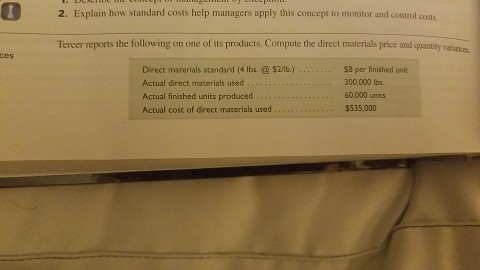# Question & Answer: 2. Explain how standard costs help managers apply this concept to monitor and control costs Tercer reports the following on one of its products. Comp…..QS 21-8 Tercer reports

Don't use plagiarized sources. Get Your Custom Essay on
Question & Answer: 2. Explain how standard costs help managers apply this concept to monitor and control costs Tercer reports the following on one of its products. Comp…..
GET AN ESSAY WRITTEN FOR YOU FROM AS LOW AS \$13/PAGE

2. Explain how standard costs help managers apply this concept to monitor and control costs Tercer reports the following on one of its products. Compute the direct materials price and quantity variun.as ces Direct materials standard ( lbs.\$2/lb.) Actual direct materials used Actual finished units produced , . … Actual cost of direct materials used. \$8 per finished unit 300,000 Ibs 60.000 urits \$535,000

Direct Materials Price Variance:

Actual Quantity = 300,000 lbs
Actual Cost = \$535,000
Standard Price = \$2 per lbs

Direct Materials Price Variance = Actual Cost – Standard Price * Actual Quantity
Direct Materials Price Variance = \$535,000 – \$2*300,000
Direct Materials Price Variance = \$535,000 – \$600,000
Direct Materials Price Variance = \$65,000 Favorable

Direct Materials Quantity Variance:

Actual Quantity = 300,000 lbs
Standard Price = \$2 per lbs

Standard Quantity per unit = 4 lbs
Standard Quantity = 4 lbs per unit * 60,000 units
Standard Quantity = 240,000 lbs

Direct Materials Quantity Variance = (Actual Quantity – Standard Quantity)*Standard Price
Direct Materials Quantity Variance = (300,000 – 240,000)*\$2
Direct Materials Quantity Variance = \$120,000 Unfavorable